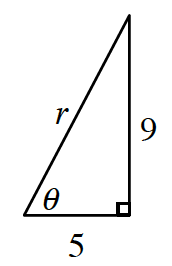### Home > PC3 > Chapter 11 > Lesson 11.1.4 > Problem11-65

11-65.

Convert the rectangular coordinates below to polar coordinates. Sketch a graph to verify that your polar coordinates lie in the correct quadrant.

1. $(5,9)$

1. $(−7,0)$

1. $(−10,3)$

Draw a diagram. The diagram below is for part (a).Use trigonometry to determine the values of $r$ and $θ$.

$5^2+9^2=r^2$                      $\tan(\theta)=\frac{9}{5}$